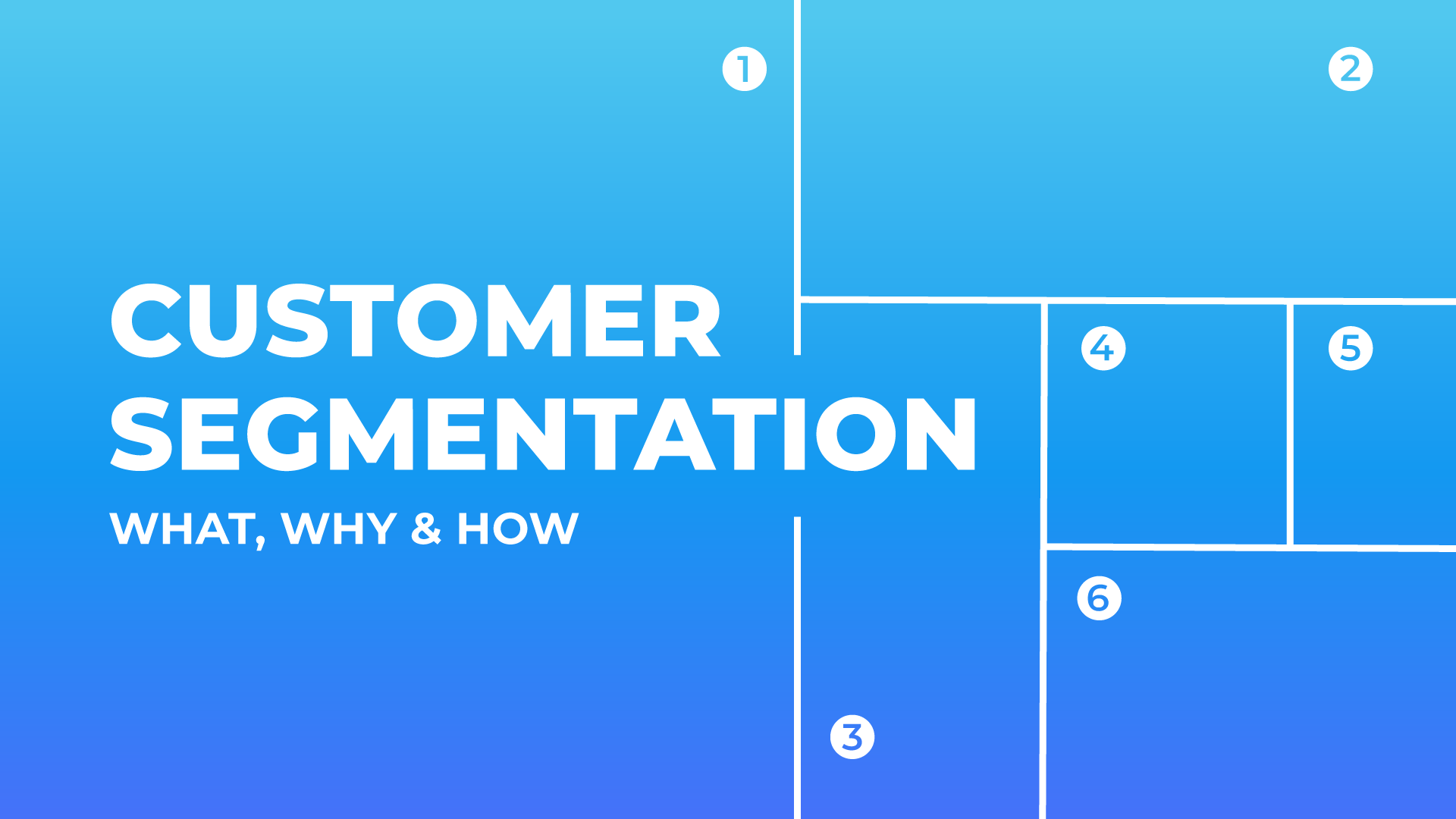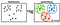# Bank Customer Segmentationhttps://www.crossengage.io/wp-content/uploads/2020/04/Segmentation-blog-post-crossengage-1.png

In my previous article, I aimed to give information about how we can simply segmentation without using any machine learning method. In this article, I will try to describe the segmentation on a dataset I found from Kaggle. But this time I’ll try to look from a different perspective using K-Means.

# Data Source

For sample analysis, I used the “Credit Card Dataset for Clustering” dataset available on Kaggle.

# Problem Statement

We have data of about 9000 credit card holders for the last 6 months. Our job is to group these customers based on their credit card usage.

# Importing Libraries and Data

`### Import libraries and Data ###import pandas as pdimport numpy as npimport seaborn as snsimport matplotlib.pyplot as pltfrom sklearn.preprocessing import StandardScaler, normalizefrom sklearn.cluster import KMeansfrom sklearn.decomposition import PCAcreditcard_df = pd.read_csv("marketing_data.csv")creditcard_df.head()creditcard_df.info()# What is the avg and max "Balance" amount?creditcard_df.agg({"BALANCE":["min","max","mean"]})creditcard_df.describe().T`

Let’s access the characteristics of the customer who makes the most “oneoff_purchases” and see when that customer makes a cash advance transaction and how often s/he pays his bill.

# EDA

First of all, we check whether there is a missing value in the data via heatmap.

`sns.heatmap(creditcard_df.isnull(), yticklabels=False, cbar=False, cmap='winter')plt.show()creditcard_df.isnull().sum()`

Fill up the missing elements with the mean of the “minimum payment” value.

`creditcard_df.loc[(creditcard_df["MINIMUM_PAYMENTS"].isnull() == True),"MINIMUM_PAYMENTS"]= creditcard_df["MINIMUM_PAYMENTS"].mean()`

Let’s fill in the missing items in the “credit_ limit” column and check again.

`creditcard_df.loc[(creditcard_df["CREDIT_LIMIT"].isnull() == True),"CREDIT_LIMIT"]= creditcard_df["CREDIT_LIMIT"].mean()creditcard_df.duplicated().sum()`

Now to plot the graphs and see what we can derive just by looking at different properties. We will do this using Distribution Plot (distplot) from matplotlib.hist and KDE Plot (kdeplot) from the seaborn library.

KDE Graph represents Kernel Density Estimate
KDE is used to visualize the Probability Density of a continuous variable.
KDE shows the probability density of different values in a continuous variable

Correlation is a method used to see the relationship between features.
A positive correlation means that the properties are directly proportional and the negative is inversely proportional, let’s now look at how the variables we have are correlated.

`# Obtain the correlationmatrix btw featuresplt.figure(figsize = (15,15))correlations = creditcard_df.corr()sns.heatmap(correlations, annot = True)plt.show()`

# K-Means

Basically, K-means is an unsupervised learning algorithm (clustering)
K-means works by grouping some data points together (clustering) in an unsupervised fashion
The algorithm groups observations with similar attribute values together by measuring the Euclidean Distance between points.https://towardsdatascience.com/k-means-a-complete-introduction-1702af9cd8c

# K-Means Algorithm Steps

1. Choose the number of clusters “K”.
2. Select random K points that are going to be the centroids for each cluster.
3. Assign each data point to the nearest centroid, doing so will enable us to create “K” number of clusters.
4. Calculate a new centroid for each cluster.
5. Reassign each data point to the new closest centroid.
6. Go to step 4 and repeat.

# How to Select The Optimal Number of Clusters (K) ?

Since the K value indicates the number of nearest neighbors. We must calculate the distances between test points and trained tag points. Updating the distance metrics every iteration is computationally difficult and slow, so we can call KNN a lazy learning algorithm.

So how is the optimum K value chosen?

There are no predefined statistical methods to find the optimal value of K.
Start a random K value and start calculating.
Choosing a small K value leads to unstable decision limits.
The significant K value is better for classification, as it leads to softening of decision boundaries.
Create a graph between the error rate in a defined range and the values representing K. The place where the angle of the line of the K value changes the most in the graph will give an idea for the optimal K value.

`scores_1=[]range_values = range(1,20)for i in range_values:    kmeans = KMeans(n_clusters= i)    kmeans.fit(creditcard_df_scaled[:,:7])    scores_1.append(kmeans.inertia_)plt.plot(scores_1, 'bx-')plt.show()`

As seen in the graph, the point where the angle changes come to somewhere between 5 and 6. Here, if we choose the value of K as 5 or 6, we will logically do the right thing.

OR

However, it wouldn’t hurt to use a library built for a more precise result. With the KElbowVisualizer function from the yellow-brick library, we can take the operations over our cluster and show us the optimum K value.

`from yellowbrick.cluster import KElbowVisualizerclusterer = KMeans()visualizer = KElbowVisualizer(clusterer, k=(2,12), metric='distortion')visualizer.fit(creditcard_df_scaled)visualizer.show()`

As we can understand from the output, the value of 5 is really the optimum k value for us.

# Apply K-Means Method

`kmeans = KMeans(5)kmeans.fit(creditcard_df_scaled)labels = kmeans.labels_kmeans.cluster_centers_.shapecluster_centers = pd.DataFrame(data = kmeans.cluster_centers_, columns=[creditcard_df.columns])cluster_centers = scaler.inverse_transform(cluster_centers)cluster_centers = pd.DataFrame(data = cluster_centers, columns = [creditcard_df.columns])cluster_centersy_kmeans = kmeans.fit_predict(creditcard_df_scaled)y_kmeanscreditcard_df_cluster = pd.concat([creditcard_df, pd.DataFrame({"cluster":labels})], axis =1)creditcard_df_cluster.head()for i in creditcard_df.columns:    plt.figure(figsize = (35,5))    for j in range(7):        plt.subplot(1,7,j+1)        cluster = creditcard_df_cluster[creditcard_df_cluster['cluster']== j]        cluster[i].hist(bins = 20)        plt.title('{}   \nCluster{}'.format(i,j))plt.show()`

# Principal Component Analysis (PCA)

PCA is an unsupervised machine learning algorithm
PCA performs dimensionality reductions while attempting at keeping the original information unchanged.
PCA works by trying to find a new set of features called components
Components are composites of the uncorrelated given input features

# Apply Principal Component Analysis and Visualize the Result

Let’s try to make it easier to understand by reducing the size with PCA.

`pca = PCA(n_components=2)principal_comp = pca.fit_transform(creditcard_df_scaled)principal_comp# Create a df with the two componentspca_df = pd.DataFrame(data = principal_comp, columns = ["pca1","pca2"])pca_df.head()`
`# Concatenate the clusters labels to the dataframepca_df = pd.concat([pca_df, pd.DataFrame({"cluster":labels})],axis = 1)pca_dfplt.figure(figsize=(10,10))ax = sns.scatterplot(x = 'pca1',y='pca2',hue="cluster", data=pca_df, palette=["red","green","blue","pink","yellow"])plt.show()`

And PCA did its job very nicely, and when we visualize the clusters formed, we can clearly select 5 separate clusters. These 5 clusters are our new segments.

I’m a Master Student🎓pursuing Data Analytics. I’m a Data Enthusiast 💻 😃 passionate about learning and working with new tech. https://github.com/ogulcanertunc

## More from Ogulcan Ertunc

I’m a Master Student🎓pursuing Data Analytics. I’m a Data Enthusiast 💻 😃 passionate about learning and working with new tech. https://github.com/ogulcanertunc